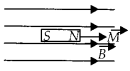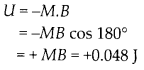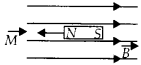Enlightened

# Question 4: NCERT Solutions for 12th Class Physics: Chapter 5-Magnetism and Matter

• 0

Question 4: NCERT Solutions for 12th Class Physics: Chapter 5-Magnetism and Matter

A short bar magnet of magnetic moment M = 0.32 J T-1 is placed in a uniform magnetic field of 0.15 T. If the bar is free to rotate in the plane of the field, which orientation would correspond to its (a) stable, and (b) unstable equilibrium? What is the potential energy of the magnet in each case?

Share

1. Solution:
(a) An equilibrium is stable, if on disturbing the magnet, it comes back to same initial state. Bar magnet is in stable equilibrium at 0 = 0° Potential energy.(b) A bar magnet is in unstable equilibrium, if on disturbing from its position, it further gets disturb and do not come back to previous position of equilibrium. At 0 = 180°, the equilibrium is unstable. Potential energyCheck the complete chapter with solutions.

NCERT Solutions for 12th Class Physics: Chapter 5-Magnetism and Matter

• 0